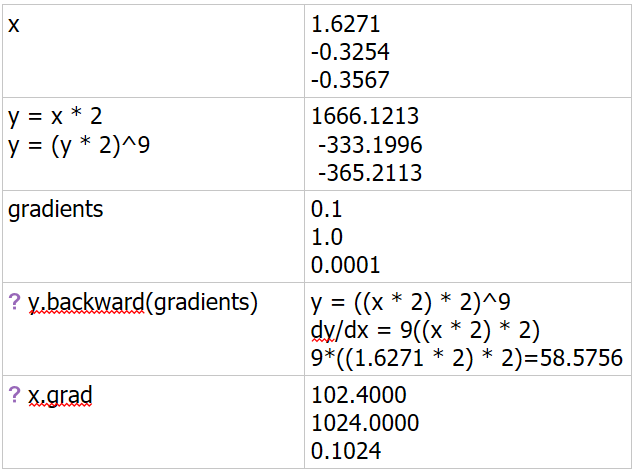# How PyTorch differentiates on non-scalar variable?

I am new to PyTorch and Neural Network in general. I am following this tutorial: http://pytorch.org/tutorials/beginner/blitz/autograd_tutorial.html#gradients

There are two examples of differentiation using `backward()`: one for scalar and one for non-scalar variable.

The scalar example:
i.stack.imgur.com/4o5qL .png

The non-scalar example:x = torch.randn(3) # input is taken randomly
x = Variable(x, requires_grad=True)

y = x * 2

c = 0
while y.data.norm() < 1000:
y = y * 2
c += 1

gradients = torch.FloatTensor([0.1, 1.0, 0.0001]) # specifying gradient because input is non-scalar

print( c )

Output:

9

102.4000
1024.0000
0.1024

I tried to understand it as I did for scalar example:

But, I can’t figure out how it works.
I get exact same output for different values of `c` regardless of the input values:

8

51.2000
512.0000
0.0512

10

204.8000
2048.0000
0.2048

I know the math behind getting `4.5000 4.5000 4.5000 4.5000` for scalar example (shown above). Can you explain the math behind getting `102.4000 1024.0000 0.1024` for non-scalar example. Please explain how it is calculating `y.backward(gradients)`

I have also asked this question on stackexchange, but no one seems to answer it:

They are plenty of sources on the forum, you should do some search before asking. For example, see discussion here

we all know the chain rule.

``````dz/dx =  dz/dy * dy/dx
``````

here
[0.1, 1.0, 0.0001 ] are dz/dy
y = 1024*x so dy/dx =1024

hope it maybe helpful

Thanks.
Could you please explain why is `y = 1024 * x`?
If `y = (y * 2)^c`, and `c = 9`, I am getting `102.4000 1024.0000 0.1024`
But, when `c = 8`, I am getting `51.2000 512.0000 0.0512`.
And when `c = 10` I am getting `204.8000 2048.0000 0.2048`
Why is that?

``````y = x*2
y = y*2
y = y*2
.....
``````

repeat it c times
and y = x*(2^(c+1))

Thank you so much!, you’re the best. I updated my solution:

1 Like